# Fractions Multiplying by more interesting fractions and then

• Slides: 27
Download presentationFractions: Multiplying by more interesting fractions – and then DIVIDING by them. (Part Two)�To find 1/8 of something, we divide that thing by 8. �What if we wanted to know what 3/8 of something was?You’d be doing the same thing 3 times, so you would multiply by 3.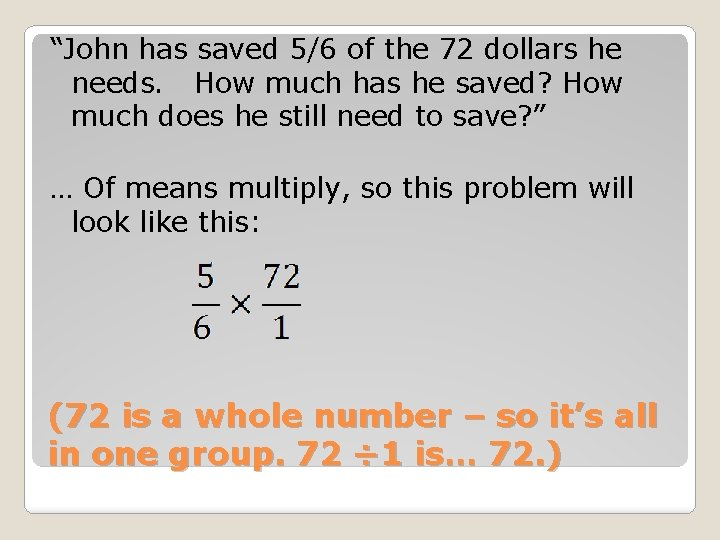“John has saved 5/6 of the 72 dollars he needs. How much has he saved? How much does he still need to save? ” … Of means multiply, so this problem will look like this: (72 is a whole number – so it’s all in one group. 72 ÷ 1 is… 72. )60 Divide 72 by 6… then multiply by 5.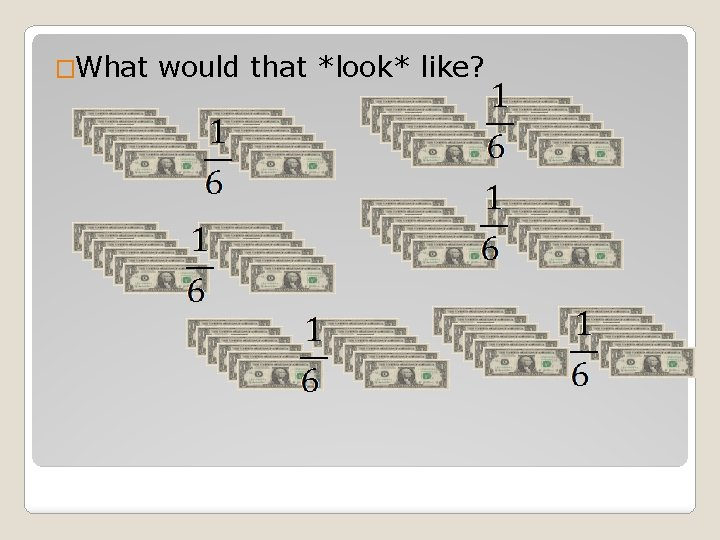�What would that *look* like?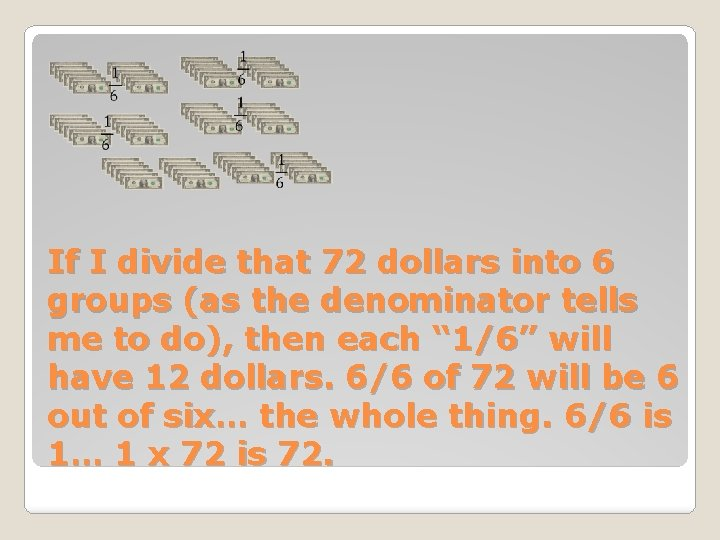If I divide that 72 dollars into 6 groups (as the denominator tells me to do), then each “ 1/6” will have 12 dollars. 6/6 of 72 will be 6 out of six… the whole thing. 6/6 is 1… 1 x 72 is 72.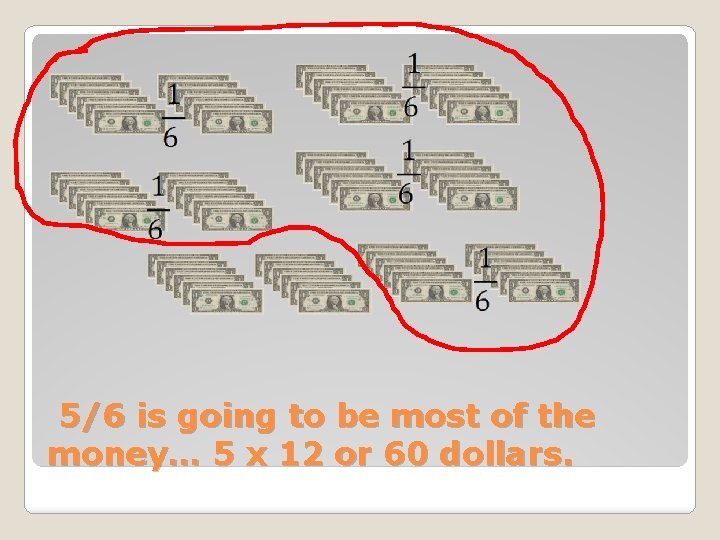5/6 is going to be most of the money… 5 x 12 or 60 dollars.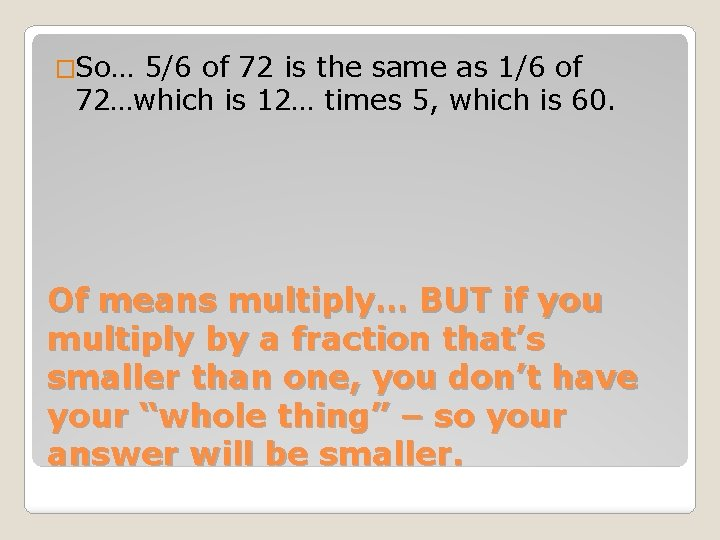�So… 5/6 of 72 is the same as 1/6 of 72…which is 12… times 5, which is 60. Of means multiply… BUT if you multiply by a fraction that’s smaller than one, you don’t have your “whole thing” – so your answer will be smaller.�How much would 3/6 of 72 be? It would be the same as ½.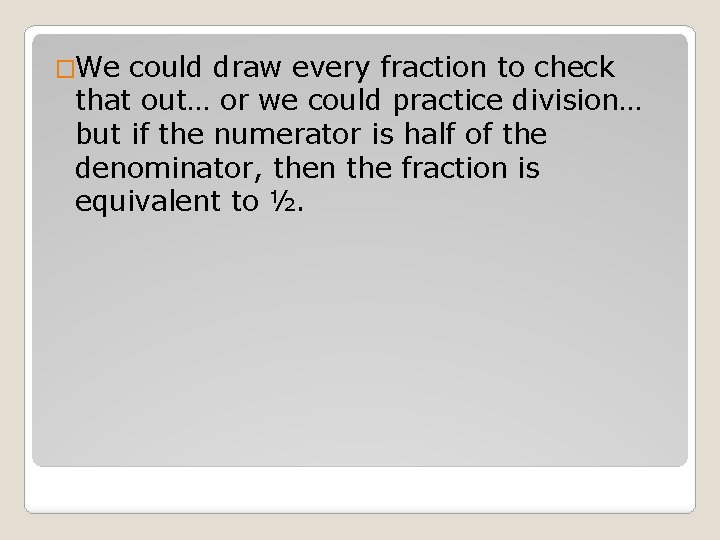�We could draw every fraction to check that out… or we could practice division… but if the numerator is half of the denominator, then the fraction is equivalent to ½.Which of these fractions are the same as a half?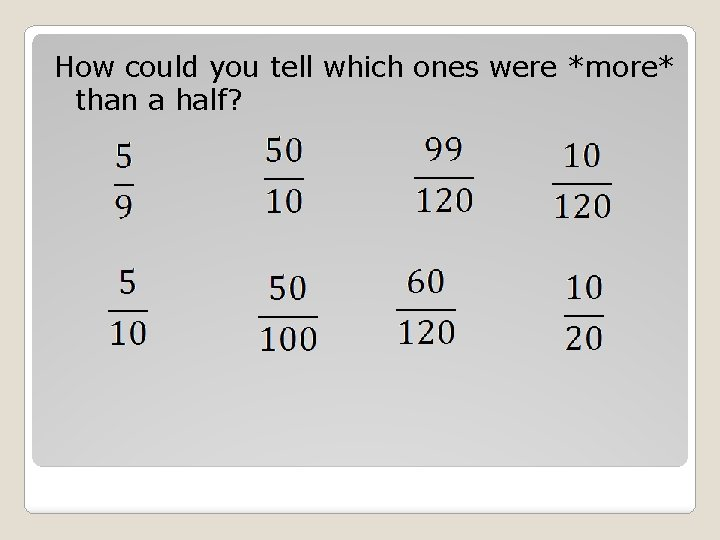How could you tell which ones were *more* than a half?�But enough with the multiplying, already… time to cover a division problem that is much easier to understand when you can see it. Dividing by fractions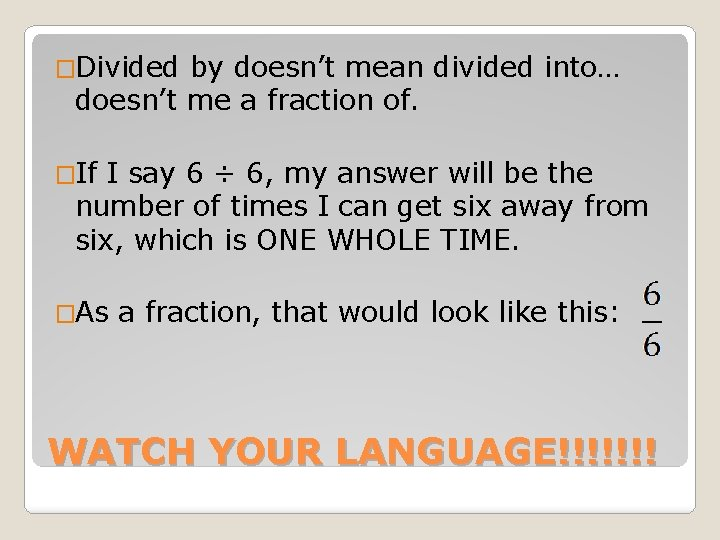�Divided by doesn’t mean divided into… doesn’t me a fraction of. �If I say 6 ÷ 6, my answer will be the number of times I can get six away from six, which is ONE WHOLE TIME. �As a fraction, that would look like this: WATCH YOUR LANGUAGE!!!!!!!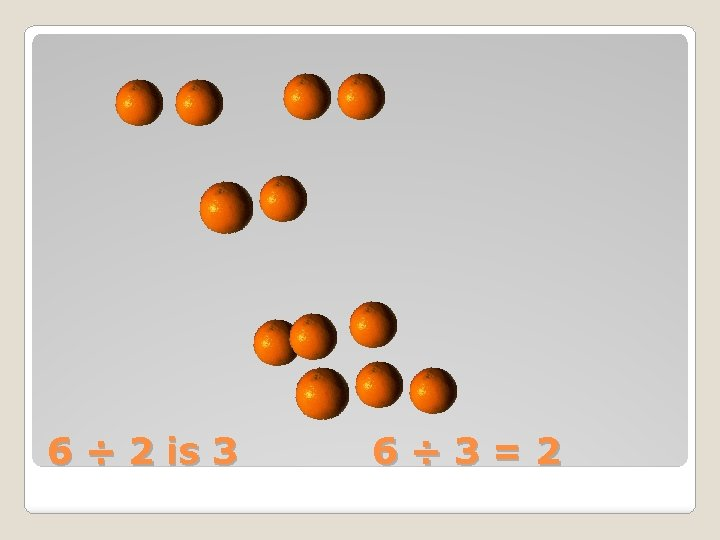6 ÷ 2 is 3 6÷ 3=2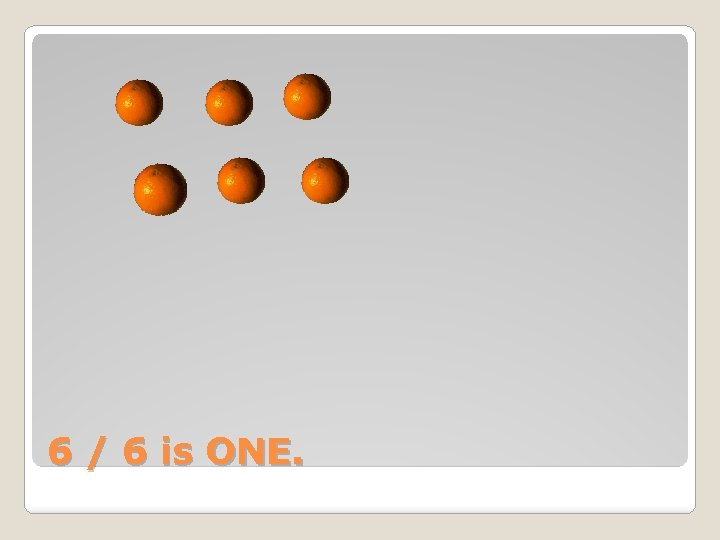6 / 6 is ONE.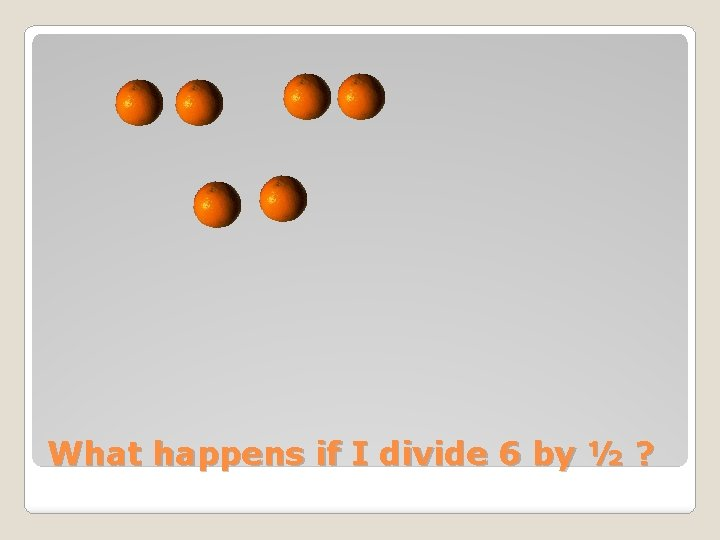What happens if I divide 6 by ½ ?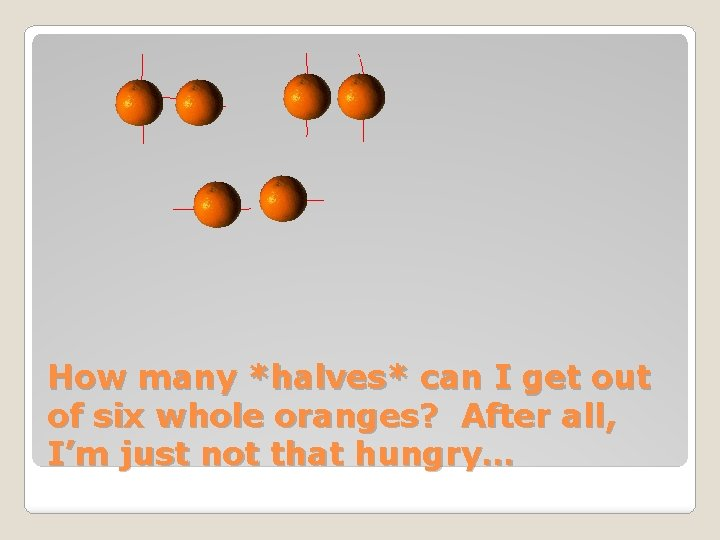How many *halves* can I get out of six whole oranges? After all, I’m just not that hungry…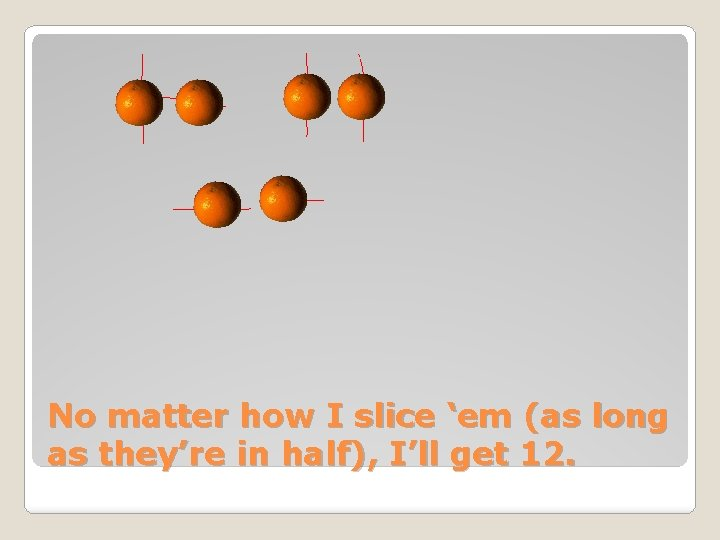No matter how I slice ‘em (as long as they’re in half), I’ll get 12.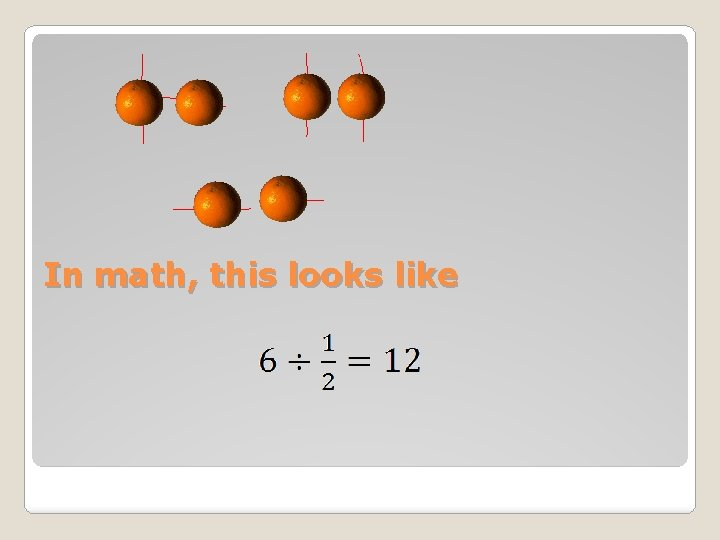In math, this looks likeAnd the way to get this without drawing everything is: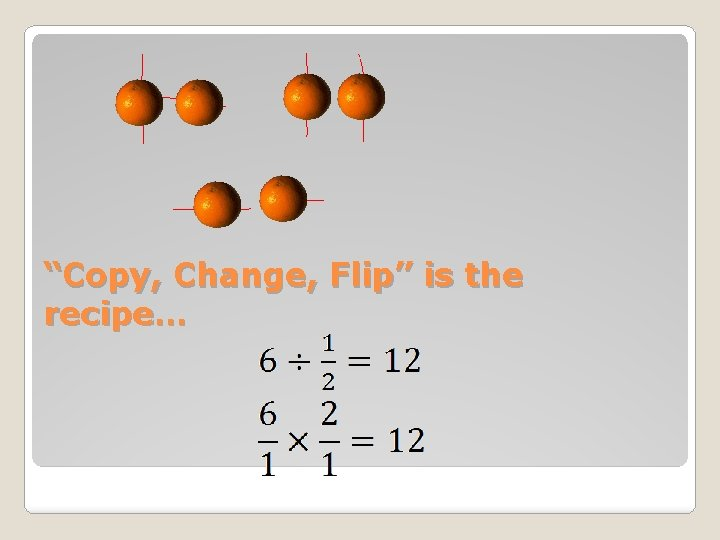“Copy, Change, Flip” is the recipe…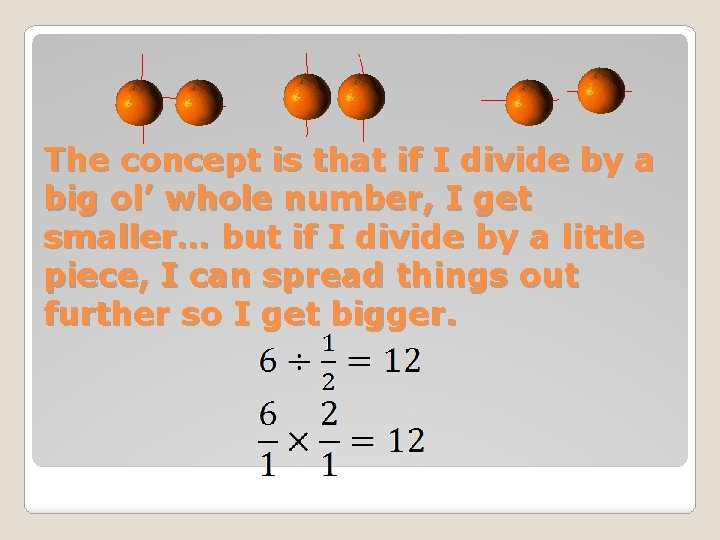The concept is that if I divide by a big ol’ whole number, I get smaller… but if I divide by a little piece, I can spread things out further so I get bigger.�You try drawing 5 ÷ 1/3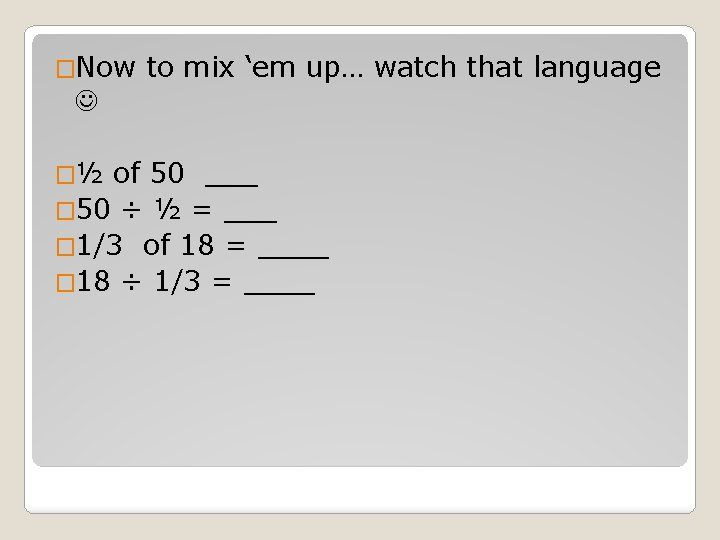�Now to mix ‘em up… watch that language �½ of 50 ___ � 50 ÷ ½ = ___ � 1/3 of 18 = ____ � 18 ÷ 1/3 = ____�Next stop… adding fractions… but don’t forget – OF means MULTIPLY